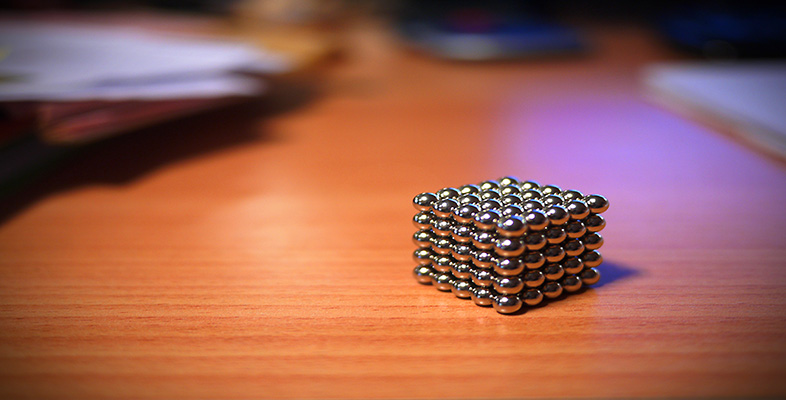Squares, roots and powers

Start this free course now. Just create an account and sign in. Enrol and complete the course for a free statement of participation or digital badge if available.

Free course

# 1.2.1 Try some yourself

## Activity 3

Without using your calculator, find the following:

• (a) 102

• (b) 1002

• (c) 0.12

• (d) 0.012

• (e)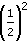• (f) (3)2

• (g) (1)2

• (a) 102 = 10 × 10 = 100

• (b) 1002 = 100 × 100 = 10 000

• (c) 0.12 = 0.1 × 0.1 =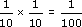= 0.01

• (d) 0.012 = 0.01 × 0.01 =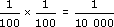= 0.0001

• (e)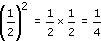• (f) (3)2 = 3 × 3 = 9

• (g) (1)2 = 1 × 1 = 1

## Activity 4

Explain why brackets should be used when writing the expression (1)2.

If you write 12 it is not clear whether you mean to square 1 first then make the answer negative, giving 1, or to square 1, giving 1. Many calculators would assume you meant (1)2, and return the answer 1.

## Activity 5

Use your calculator to find the following, using your answers to the appropriate parts of Question 1 as estimates.

• (a) 962

• (b) 10.652

• (a) Rough check: 1002 = 10 000.

Calculator gives 962 = 9216.

• (b) Rough check: 102 = 100.

Calculator gives 10.652 = 113.4225.

MU120_4M4